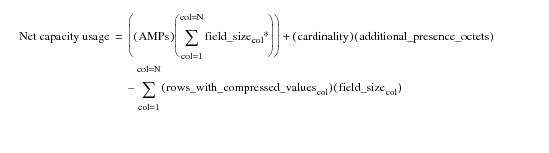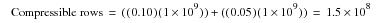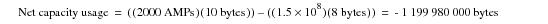# 15.00 - Detailed Calculation of Multi-Value Net Capacity Usage - Teradata Database

prodname
vrm_release
15.00
category
User Guide
featnum
B035-1094-015K

### Detailed Calculation of Multi-Value Net Capacity Usage

“Equation 7: Net Capacity Usage for Multi-Value Compression With Fallback Not Enabled” on page 727 and “Equation 8: Net Capacity Usage for Multi-Value Compression With Fallback Enabled” on page 728 describe net capacity usage at a high-level. “Equation 12: Multi-Value Net Capacity Usage” presents the same formula in greater detail.

#### Equation 12: Multi-Value Net Capacity Usagewhere:

 This term … Specifies the number of … N compressed columns in the table. AMPs AMPs in the system. field_sizecol* the number of table header bytes used by the compressed column + 1. cardinality rows in the table. additional_presence_octets presence octets in the table determined by “Equation 9: Additional Presence Octets” on page 728. rows_with_compressed_values rows in the table having compressed values. field_sizecol bytes in the compressed column.

### Example Multi-Value Net Capacity Usage Calculation

With the information provided by equations 1, 2, 3, and 4, you are now able to calculate the net capacity usage for a table. This example is for single‑valued compression. Consider the following table demographics.

 Characteristic Description Cardinality 1 x 109 rows Candidate column Base column size is 8 bytes (`CHARACTER(8)`) Calculated column size is 10 bytes (see “field_sizecol” in the preceding table). column is nullable 10% of rows have a common value for the column 5% of rows are null for the column Number of AMPs in system 2,000 Additional presence octets 0 Only one column is compressed. Number of rows having compressed columnThe value for net capacity usage is calculated as follows. Note that because there is only 1 presence octet, the row_header_space term evaluates to 0, so it has no effect in the computation.The highly negative result indicates that the column is an excellent candidate for compression.